# Edexcel A Level Chemistry:复习笔记1.7.1 Reacting Volume Calculations

### Reacting Volume Calculations

• Reacting volume calculations are commonly associated with the reactions of gases
• All gases occupy the same volume under the same conditions
• At room temperature and pressure (r.t.p.), the molar gas volume of any gas is 24.0 dm3
• Room temperature and pressure are 293 K / 20 oC and 101.3 kPa respectively
• At standard temperature and pressure (s.t.p.), the molar gas volume of any gas is 22.4 dm3
• Standard temperature and pressure are 273 K / 0 oC and 101.3 kPa respectively
• The equation to calculate the number of moles for any volume of gas is:

Number of moles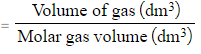#### Worked Example

Molar gas volume calculations

Calculate the number of moles present in 4.5 dm3 of carbon dioxide at:

1. Room temperature and pressure
2. Standard temperature and pressure

1. Number of moles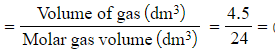0.1875 moles
2. Number of moles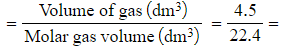0.201 moles (to 3 s.f.)
• Volumes of gas can also be calculated using
• Number of moles calculations
• Volume calculations
• This can help determine the size of the equipment that you use in an experiment

#### Worked Example

Calculating gas volumes from moles

Calculate the volume of gas produced when 1.50 g of sodium reacts with water at standard temperature and pressure.

Step 1: Write the balanced equation for the reaction

• 2Na (s) + H2O (l) → 2NaOH (aq) + H2 (g)

Step 2: Calculate the number of moles of sodium

• Number of moles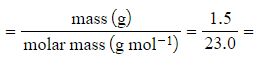0.0652 moles (to 3s.f.)

Step 3: Use the stoichiometry of the equation to calculate the number of moles of hydrogen

• The stoichiometric ratio of Na : H2 is 2 : 1
• Therefore, the number of moles of hydrogen is0.0326 moles

Step 4: Calculate the volume of hydrogen gas evolved

• Volume of gas = number of moles x molar gas volume
• Volume of gas = 0.0326 x 22.4 = 0.730 dm3 (to 3s.f.)

#### Worked Example

Calculating gas volumes from other volumes

Calculate the total volume of gas produced when 6.50 dm3 of propane combusts completely

Step 1: Write the balanced equation for the reaction

• C3H8 (g) + 5O2 (g) → 3CO2 (g) + 4H2O (g)

Step 2: Determine the number of moles of gas produced

• One mole of propane produces 3 moles of carbon dioxide and 4 moles of water
• Therefore, one mole of propane produces a total of 7 moles of gas

Step 3: Calculate the volume of gas that is produced

•  6.50 dm3 of propane will produce 7 x 6.50 dm3 of gas = 45.5 dm3 gas

### Ideal Gases

#### Kinetic theory of gases

• The kinetic theory of gases states that molecules in gases are constantly moving
• The theory makes the following assumptions:
• That gas molecules are moving very fast and randomly
• That molecules hardly have any volume
• That gas molecules do not attract or repel each other (no intermolecular forces)
• No kinetic energy is lost when the gas molecules collide with each other (elastic collisions)
• The temperature of the gas is related to the average kinetic energy of the molecules

• Gases that follow the kinetic theory of gases are called ideal gases
• However, in reality gases do not fit this description exactly but may come very close and are called real gases

#### Ideal gas equation

• The ideal gas equation shows the relationship between pressure, volume, temperature and number of moles of gas of an ideal gas:

#### PV = nRT

• P = pressure (pascals, Pa)
• V = volume (m3)
• n = number of moles of gas (mol)
• R = gas constant (8.31 J K-1 mol-1)
• T = temperature (kelvin, K)

#### Worked Example

Calculating the volume of a gas

Calculate the volume occupied by 0.781 mol of oxygen at a pressure of 220 kPa and a temperature of 21 °C

Step 1: Rearrange the ideal gas equation to find volume of gas

V =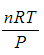Step 2: Calculate the volume the oxygen gas occupies

• P = 220 kPa = 220 000 Pa
• n = 0.781 mol
• R = 8.31 J K-1 mol-1
• T = 21 oC = 294 K

V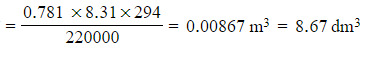#### Worked Example

Calculating the molar mass of a gas

A flask of volume 1000 cm3 contains 6.39 g of a gas. The pressure in the flask was 300 kPa and the temperature was 23 °C.

Calculate the relative molecular mass of the gas.

Step 1: Rearrange the ideal gas equation to find the number of moles of gas

n =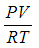Step 2: Calculate the number of moles of gas

• P = 300 kPa = 300 000 Pa
• V = 1000 cm3 = 1 dm3 = 0.001 m3
• R = 8.31 J K-1 mol-1
• T = 23 oC = 296 K

n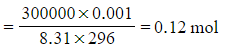Step 3: Calculate the molar mass using the number of moles of gas

n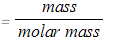molar mass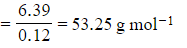#### Exam Tip

To calculate the temperature in Kelvin, add 273 to the Celsius temperature, e.g. 100 oC is 373 Kelvin.

You must be able to rearrange the ideal gas equation to work out all parts of it.

The units are incredibly important in this equation - make sure you know what units you should use, and do the necessary conversions when doing your calculations!